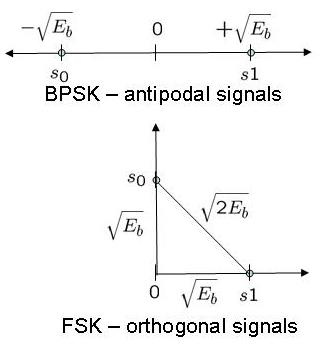# Bit Error Rate (BER) for frequency shift keying with coherent demodulationFollowing the request by Siti Naimah, this post discuss the bit error probability for coherent demodulation of binary Frequency Shift Keying (BFSK) along with a small Matlab code snippet.

Using the definition provided in Sec 4.4.4 of [DIG-COMM-SKLAR]), in binary Frequency shift keying (BFSK), the bits 0’s and 1’s are represented by signals and having frequencies and respectively, i.e.

,

where

is the energy ,

is the symbol duration and

is an arbitrary phase (assume to be zero).

The two frequencies and are orthogonal, i.e.

and

.

Simple transmit-receive block diagram for binary frequency shift keying (FSK) can be as shown below.Figure: Block diagram of FSK modulation and coherent demodulation

For analyzing the bit error rate with coherent FSK demodulation, let us compare the signaling waveform used by binary FSK when compared with binary PSK. The distance between the energy of the signaling waveform for:

(a) binary phase shift keying (BPSK) is (uses antipodal signaling)

(b) binary frequency shift keying (BFSK) is (uses orthogonal signaling)Figure: Orthogonal and antipodal signaling

Using similar mathematical formulation used for BPSK, but with the distance between the signals reduced by half, the bit error probability for coherent binary frequency shift keying is

.

For obtaining the same bit error rate as BPSK, binary frequency shift keying requires around 3dB more . More details on the bit error curves with orthogonal and antipodal signals is discussed in Sec 3.2.5 of [DIG-COMM-SKLAR].

## Simulation Model

Simple Matlab/Octave script for computing the bit error rate with FSK modulation. The code performs the following:

(a) Generation of random 1’s and 0’s

(b) Converting bits to appropriate frequency

(c) Passing through Additive White Gaussian Noise channel

(e) Counting the number of errors.Figure: Bit error probability with coherent demodulation of frequency shift keying

Hope this helps

Krishna

Reference

[DIG-COMM-SKLAR] Digital Communications: Fundamentals and Applications (2nd Edition), Bernard Sklar## 22 thoughts on “Bit Error Rate (BER) for frequency shift keying with coherent demodulation”

1.Ramya says:

what kind of method did you use to find the bit error rate?
how does it vary if I am supposed find out BER by using monte-carlo iterations?

1.Krishna Sankar says:

@Ramya: For find the bit error rate, compare the received and transmitted bits and count the number of errors. Number of errors by total number of bits is the error rate

2.Siddharth says:

I am trying to code 16-FSK modulator and then compute average energy.How can I do this? Your help will be appreciated.

1.Krishna Sankar says:

@Siddharth: Define your signal vector and find the mean square power.

3.zardosht says:

Hi krishna
I cant understand number of symbole, where is
i want some information about it.

1.Krishna Sankar says:

@zardosht: Hmm… the k = log2(M) means, if we have M constellation points we can send k = log2(M) bits in a constellation symbol. For eg, with 16QAM we can send 4 bits together.

4.Mahadevan Srinivasan says:

Hi Krishna,

I recently found this website. Great Effort. :).

5.Chandrakanth says:

Can someone help me out on this please. I am looking for MAT LAB PROGRAM TO IMPLEMENT HUFFMAN CODING.

Thank you

1.Krishna Sankar says:

@Chandrakanth: Sorry, I have not discussed Huffman coding till date.

6.Srinivas says:

Can someone help me out on this please.
For ISI channel with L real coeffs and BPSK modulation write a Viterbi algorithm decoder with decoding delay >0
Using Matlab
Plot BER vs SNR for varying delays

I need the matlab code.
Thank You

1.Krishna Pillai says:

@Srinivas: I have not done MLSE equalization in multipath channel, but plan to do that in near future.

7.RAO says:

hi krishna,

i want to plot the BER vs Eb/No of fsk coherent and noncoherent on awgn and raleigh channels.
can u help me in this regard?

1.Krishna Pillai says:

@RAO: Sorry, I have not tried modeling FSK with non-coherent demodulation OR in Rayleigh channel.

8.Joe says:

What will BER be for M-FSK, if M=4?

1.Krishna Pillai says:

@Joe: Am not sure about the precise answer, however a quick googling threw up the following link.
Noise Performances of different Modulation Schemes – Chris Haji-Michael
Hope this is useful.

9.kalfika says:

Hi Krishna.
Your simulation is very useful for me.
Krishna, I want to ask about continuous fsk. Is continuous phase FSK same with fsk? How we define continuous phase in fsk?

1.kalfika says:

Can we use MSK to modulate 4-ary or 8-ary? Because I think MSK is just to modulate binary. What do you think about that?

1.Krishna Pillai says:

@kalfika: Well, am also not aware of MSK systems using 4-ary/8-ary. Quick googling did not fetch me much results.

10.mohit says:

hii

11.Krishna Pillai says:

@davis: Well, given that we need to send both 1Hz and 2Hz, I do not think that the data bandwidth becomes equal to the carrier separation. Given the above understanding, am unable to understand your perspective. Can you please provide more details.

12.davis says:

Hi Krishna
i see that in the code the freqency for 0 is 1 Hz
and for 1 2 Hz
and that bit period is 1sec witch means that the data band width is the same as the seperation between the to carriers
How is this possible?
Thanks